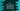# std::reverse() method in C++ explanation with example## std::reverse() method in C++:

std::reverse() is defined in the algorithm header file. It is used to reverse the order of elements in a given range.

In this post, we will learn how to use std::reverse with example.

### Syntax of std::reverse:

std::reverse is defined as below:

``void reverse (BidirectionalIterator first, BidirectionalIterator last);``

It takes two parameters first and last. These are bidirectional iterator to the initial and final positions of the sequence.

It reverses the order of the elements in the index range of [first, last). [first, last) defines that the reversing process considers all the elements from index first to last, including the element at index first, but excluding the element at index last.

### How to reverse a string by using std::reverse:

We can also use std::reverse to reverse a string in C++. We need to pass the start and end character index of the string to work this with std::reverse. Below is the complete example:

``````using namespace std;
#include <iostream>
#include <string>
#include <algorithm>

int main()
{
string firstStr = "Hello World !!";
string secondStr = "1234567";
string thirdStr = "****^^^^%%%%";
string fourthStr = "H e l l o";

reverse(firstStr.begin(), firstStr.end());
reverse(secondStr.begin(), secondStr.end());
reverse(thirdStr.begin(), thirdStr.end());
reverse(fourthStr.begin(), fourthStr.end());

cout << firstStr << endl;
cout << secondStr << endl;
cout << thirdStr << endl;
cout << fourthStr << endl;

return 0;
}``````

Here,

• We need to include header.
• This program is testing four different strings, firstStr, secondStr, thirdStr and fourthStr.
• reverse method is reversing all of these strings. The index of the first character is passed as the first parameter and the position next to the last character is passed as the second parameter. For these positions, begin() and end() methods are used respectively.
• Once the reverse is done, it changes the content of the strings. The last four cout lines are printing these updated values.

If you run this app, it will print the below output:

``````!! dlroW olleH
7654321
%%%%^^^^****
o l l e H``````

As you can see here, all strings are reversed.

You can also try with different strings to check how it works.